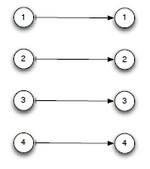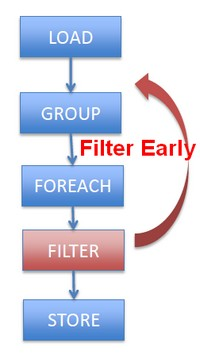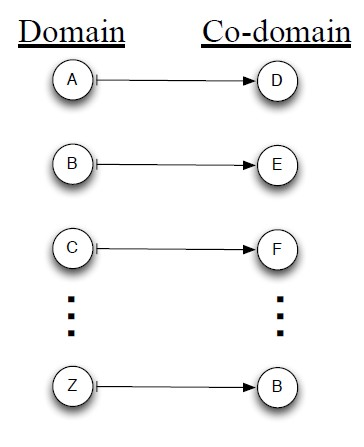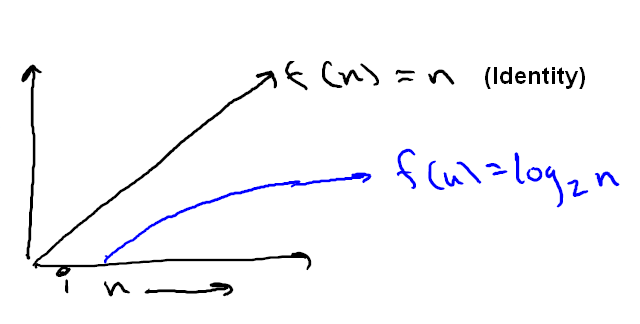# Function - Identity

An identity function is an algebraic law that defines when the output of the function is equal to the input.

## Linear Algebra

For any domain D: each domain element d map to itself:

 $id_D : D \rightarrow D$## Example

Addition (+) $$x+0 = x$$

Division: (/) $$\frac{x}{1} = x$$

Recommended Pages(Function|Operator) - Algebraic (Laws|properties) - Axioms

Property of function and operator Continuous SmoothAlgebraic Optimization

Same as: Algebraic Laws: (+) identity: x+0 = x (/) identity: x/1 = x () distributes: (nx+ny) = n(x+y) () commutes: xy = yx Apply rules 1,3,4,2 and we get Two operations instead of five,...Linear Algebra - Function (Set)

For each input element in a set , a function assigns a single output element from another set . is called the domain of the function is called the co-domain or in Mathese: The function...Mathematics - Inverse axiom

/ is inverse of - is inverse of + root is the inverse of exponentiation Functions f and g are functional inverses if: and are defined and are functions.Mathematics - Logarithm Function (log)

is the number (#) of times you divide n by b until you get down to 1. trees With the base 2 where is the # of times you divide n by 2 until you get down to 1. You keep repeating dividing by two...Number - Addition (or Sum, Sigma) or Total

The addition of all numbers in a set is called a total. Sum can be use as: an aggregate or a analytic function. It can follow the full syntax of the analytic function and in this way create It's...Number - Division

A division is a mathematical binary function between 2 numbers. It is the definition of every rational number. where: The division symbol ÷ is just a blank fraction with dots replacing...Trignometry - Identity function (sin2+cos2=1)

identify function in Trignometry where: Other illustration : The identity function is a derivative from the pythagorean...